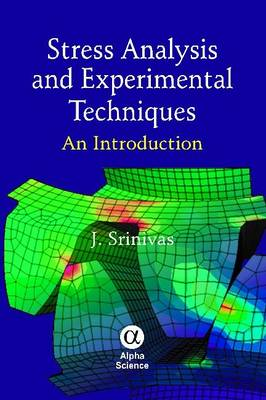•Stress Analysis and Experimental Techniques: An Introduction (Hardback)

(author)
£39.95
Hardback 366 Pages / Published: 02/12/2011
• We can order this

Usually dispatched within 1 week

STRESS ANALYSIS AND EXPERIMENTAL TECHNIQUES: An Introduction covers the basic needs of engineers working in the area of stress-analysis, important concepts of theoretical and experimental techniques in stress-analysis are explained in simple chapters. Concepts of fundamental solid mechanics such as shear force, bending moment, stress and deflection analysis of beams, torsion of circular and noncircular shafts, stability analysis of columns and stress analysis of thick and compound cylindrical shells are initially presented. Basic finite element analysis concepts needed for stress-analysis are introduced. Conventional experimental techniques like photoelasticity, moire-fringe analysis, strain gauge approach and brittle-coating methodology are elucidated in simple terms. In summary, the book Includes a good number of numerical examples Offers solution methods to several static and dynamic problems in stress analysis Provides a number of references and web-resources Gives basic hints to conduct case studies using experimental stress analysis techniques

Publisher: Alpha Science International Ltd
ISBN: 9781842657232
Number of pages: 366
Dimensions: 240 x 160 mm